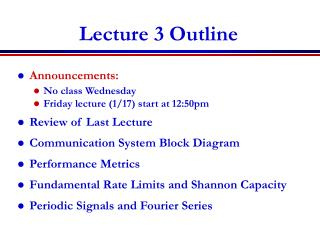DownloadDownload PresentationLecture 3 Outline

# Lecture 3 Outline

Télécharger la présentation## Lecture 3 Outline

- - - - - - - - - - - - - - - - - - - - - - - - - - - E N D - - - - - - - - - - - - - - - - - - - - - - - - - - -
##### Presentation Transcript

1. Lecture 3 Outline • Announcements: • No class Wednesday • Friday lecture (1/17) start at 12:50pm • Review of Last Lecture • Communication System Block Diagram • Performance Metrics • Fundamental Rate Limits and Shannon Capacity • Periodic Signals and Fourier Series

2. Review of Last Lecture • Analog, digital, and binary signals • Analog communication systems • Convert analog information signals to modulated analog signals • Digital communication systems • Convert bits to modulated digital signals • Communication system block diagram

3. Transmitter Communication System Block Diagram Text Images Video • Source encoder converts message into message signal or bits. • Transmitter converts message signal or bits into format appropriate for channel transmission (analog/digital signal). • Channel introduces distortion, noise, and interference. • Receiver decodes received signal back to message signal. • Source decoder decodes message signal back into original message. Source Encoder Source Decoder Channel Receiver

4. Performance Metrics • Analog Communication Systems • Metric is fidelity • Want m(t)m(t) • Digital Communication Systems • Metrics are data rate (R bps) and probability of bit error (Pb=p(bb)) • Without noise, never make bit errors • With noise, Pb depends on signal and noise power, data rate, and channel characteristics. ^ ^

5. Data Rate Limits • Data rate R limited by signal power, noise power, distortion, and bit error probability • Without distortion or noise, can have infinite data rate with Pb=0. • Shannon capacity defines maximum possible data rate for systems with noise and distortion • Rate achieved with bit error probability close to zero • In white Gaussian noise channels, C=B log(1+Ps/PN) • Does not show how to design real systems • Shannon obtained C=32 Kbps for phone channels • Get 1.5 Mbps with DSL by using more bandwidth

6. -T0 0 T0 2T0 Periodic Signals • xp(t) periodic if exists T such that xp(t)=xp(t+T) for all t. • Smallest such T is fundamental period T0 • Any integer multiple of T0 is a period of xp(t) • Fundamental period defined as f0=1/T0 • Aperiodic signals are not periodic

7. -T0 0 T0 2T0 0 T0 Projection of Signals • Fourier series representation • Project periodic signals onto basis functions • Periodic signal is weighted sum of these functions c2 c1 c3 0 0 T0 T0

8. Exponential Basis Functions • Fourier series uses exponential basis fcns • Fourier series representation • The {cn}s are the Fourier Series coefficients • These represent the frequency components of the periodic signal.

9. Main Points • Transmitter converts information into a signal appropriate for transmission, receiver does reverse. • Performance metric for analog systems is fidelity, for digital it is rate and error probability. • Data rates over channels with noise have a fundamental capacity limit. • Fourier series represents periodic signals as a weighted sum of exponential functions. • The Fourier series coefficients are the frequency components of the periodic signal.